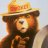# How to: indicator to draw horiz bars across day, week, month

R

#### rlewkov

##### New member
Hello,
Rookie TOS scripter here. I have a couple of things I'd like to understand and seeing an example may help.
I want to understand a) drawing of lines across day/week/month range b) how to access data at different levels of granularity in the same indicator c)
date arithmetic in TOS. This indicator example will help me understand these things.

If this is too big of a “question” I understand and if this would be better asked in a different forum please let me know.

Overview
Depending on the timeframe of data I'm viewing (intraday or daily) I'd like to draw horizontal lines above/below a certain range of bars
Description
• Assume uppercase variables are inputs to the script
• Assume PctRange(aDate, NBAR, PCT) will compute a percentage of the average range (H-L) of the past NBAR starting at aDate. E.g. compute 5 percent of the 10 bar average range starting on Aug 10, 2019
Indicator Definition
Based on the timeframe of data being viewed I'd like an indicator to draw:
• Intraday: Draw horizontal bars across the day that is PCtRange() above/below the first NMIN of the day. E.g., Draw horizontal bar +-PctRange(today-1, NBAR, PCT) above/below the first 10 min (NMIN) range of the day
• Daily(1): Draw horizontal bars across the week that is PCtRange() above/below the first NMIN of the first day of the week.
• E.g., Draw horizontal bar +-PctRange(firstDayOfWeek-1, NBAR, PCT) above/below the first 60 min (NMIN) range of the first day of the week. The bars would extend from first to last day of week
• Daily(2): Draw horizontal bars across the month that is PCtRange() above/below the first NMIN of the first day of the month
• E.g., Draw horizontal bar +-PctRange(firstDayOfMonth -1, NBAR, PCT) above/below the first day of the month The bars would extend from first to last day of monthMidpoint Moving Average Indicator Questions 2